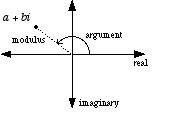index: click on a letter A B C D E F G H I J K L M N O P Q R S T U V W X Y Z A to Z index index: subject areas numbers & symbols sets, logic, proofs geometry algebra trigonometry advanced algebra & pre-calculus calculus advanced topics probability & statistics real world applications multimedia entrieswww.mathwords.com about mathwords website feedback

 Argument of a Complex Number Polar Angle of a Complex Number The angle describing the direction of a complex number on the complex plane. The argument is measured in radians as an angle in standard position. For a complex number in polar form r(cos θ + isin θ) the argument is θ.See also